Important Questions Class 7 Maths Chapter 6: Triangle and Its Properties# Triangle and Its Properties Questions

In this page we have Important Questions Class 7 Maths Chapter 6: Triangle and Its Properties . Hope you like them and do not forget to like , social share and comment at the end of the page.

## True and False Statement

Question 1.
(a) The interior of a triangle includes its vertices.
(b) The triangular region includes the vertices of the corresponding triangle.
(c) An equilateral triangle is isosceles also.
(d) The sum of the measures of three angles of a triangle is greater than 180°
(e) It is possible to have a triangle in which two of the angles are obtuse.
(f) It is possible to have a triangle in which two angles are acute.
(g) It is possible to have a triangle in which each angle is less than 60°.
(h) It is possible to have a triangle in which each angle is greater than 60°
(i) In a triangle Sum of any two sides of a triangle is not less than the third side.
(j) In a triangle, sum of squares of two sides is equal to the square of the third side.

(a) T
(b) T
(c) T
(d) F
(e) F
(f) T
(g) F
(h) F
(i) F
(j) F

## Fill in the blanks

Question 2.
(a) If one side of a triangle is produced, the exterior angle so formed is equal to the sum of its ____________________.
(b) A triangles has ____________ elements.
(c) The sum of any two sides of a triangle is _______________________ than third side.
(d) The difference of any two sides of a triangle is ____________ than side.
(e) The ________triangle always has altitude outside itself.
(f) In the below figure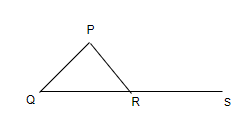$\angle PRS = \angle QPR + \angle$ ________.
(g) In an isosceles triangle, two angles are always ____________.

(a) Oppossite Interior angles
(b) 6
(c) greater
(d) less
(e)Obtuse
(f) $\angle PQR$
(g) equal

## Multiple Choice Questions

Question 3.
In $\Delta PQR$,
(a) PQ - QR > PR
(b) PQ + QR < PR
(c) PQ - QR< PR
(d) PQ + PR< QR

Question 4.
In an isosceles triangle, one angle is 50°. The other two angles are of
(i) 65° and 65° (ii) 50° and 80° (iii) any measure
In the given option(s) which of the above statement(s) are true?
(a) (i) only
(b) (ii) only
(c) (iii) only
(d) (i) and (ii)

Question 5.
Which of the following triplets cannot be the angles of a triangle?
(a) 67°, 51°, 62°
(b) 70°, 83°, 27°
(c) 90°, 70°, 20°
(d) 40°, 132°, 18°

Question 6.
In a right-angled triangle ABC, if angle C = 90°, then which of the following is true?
(a) $AB^2 = BC^2 + AC^2$
(b) $AC^2 = AB^2 + BC^2$
(c) AB = BC + AC
(d) AC = AB + BC

Question 7.
How many altitudes does a triangle have?
(a) 1
(b) 6
(c) 3
(d) 9

Question 8.
Which of the following statements is not correct?
(a) The sum of any two sides of a triangle is greater than the third side
(b) A triangle can have all its angles acute
(c) A right-angled triangle cannot be equilateral
(d) Difference of any two sides of a triangle is greater than the third side

3. (c)
4. (d)
5. (d)
6. (a)
7. (c)
8. (d)

Question 9.
An iron rod 5m long is placed against the wall in such a way that the foot of the rod is 3m away from the wall. Find how high the top of the iron rod reaches in the wall ?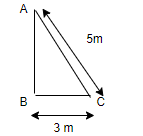$AC^2 = BC^2 + AB^2$
$25=AB^2 +9$
$AB^2 =16$
AB=4 m

Question 10.
One of the exterior angles of a triangle is 120° and the interior opposite angles of it are in the ratio 4 : 2. Find the angles of the triangle.

Let the angles be 4x and 2x
Then
4x +2 x=120
x= 20
So angles are 80°,40°,60°

Question 11.
Here in the given figure $\angle BAC=90^0$ , $\angle ABC =65^0$, $AD \perp BC$. Find p and q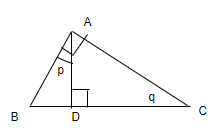In $\Delta ABC$
$\angle ABC + q + 90 =180$
$q= 90-65= 25^0$
In $\Delta ABD$
$\angle ABD + p + 90 =180$
$p=25^0$

Question 12.
From the adjoining figure, find the value of x and also the measures of $\angle P$,$\angle Q$ and $\angle R$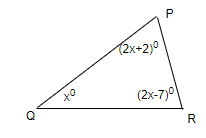$2x+2 +x + 2x-7=180$
$5x=185$
$x=37^0$
So angles are 76°, 37°,67°

Question 13.
Find the value of a,b and c in the below figures
(i)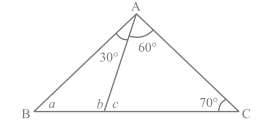(ii)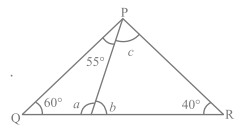(i) In $\Delta ABC$
$a + 90 + 70=180$
a=20°
Also
$a + 30 + b=180$
b= 130°
ALso
$60 + c + 70=180$
c=50°
(ii) In $\Delta ABC$
$55 + c + 60 + 40 =180$
c= 25°
Now
$25 + b + 40=180$
b= 115°
Now
$a + 60 + 55=180$
a=65°

Question 14
find the measures of $\angle x$ and $\angle y$.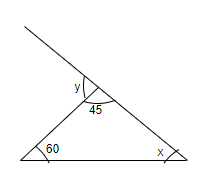First
60 + 45 + x=180
x= 75°
Now
y=x+ 60
=75 + 60 = 135°

Assignments